# Time-Dependent System Reliability (Analytical)

(diff) ← Older revision | Latest revision (diff) | Newer revision → (diff)

New format available! This reference is now available in a new format that offers faster page load, improved display for calculations and images, more targeted search and the latest content available as a PDF. As of September 2023, this Reliawiki page will not continue to be updated. Please update all links and bookmarks to the latest reference at help.reliasoft.com/reference/system_analysis

 Chapter 4: Time-Dependent System Reliability (Analytical)

In the RBDs and Analytical System Reliability chapter, different system configuration types were examined, as well as different methods for obtaining the system's reliability function analytically. Because the reliabilities in the problems presented were treated as probabilities (e.g., $\displaystyle P(A)\,\!$ , $\displaystyle {{R}_{i}}\,\!$ ), the reliability values and equations presented were referred to as static (not time-dependent). Thus, in the prior chapter, the life distributions of the components were not incorporated in the process of calculating the system reliability. In this chapter, time dependency in the reliability function will be introduced. We will develop the models necessary to observe the reliability over the life of the system, instead of at just one point in time. In addition, performance measures such as failure rate, MTTF and warranty time will be estimated for the entire system. The methods of obtaining the reliability function analytically remain identical to the ones presented in the previous chapter, with the exception that the reliabilities will be functions of time. In other words, instead of dealing with $\displaystyle {{R}_{i}}\,\!$ , we will use $\displaystyle {{R}_{i}}(t)\,\!$ . All examples in this chapter assume that no repairs are performed on the components. Repairable systems analysis will be introduced in a subsequent chapter.

## Analytical Life Predictions

The analytical approach presented in the prior chapter involved the determination of a mathematical expression that describes the reliability of the system, expressed in terms of the reliabilities of its components. So far we have estimated only static system reliability (at a fixed time). For example, in the case of a system with three components in series, the system's reliability equation was given by:

$\displaystyle {{R}_{s}}={{R}_{1}}\cdot {{R}_{2}}\cdot {{R}_{3}} \ \,\!$

The values of $\displaystyle {{R}_{1}}\,\!$ , $\displaystyle {{R}_{2}}\,\!$ and $\displaystyle {{R}_{3}}\,\!$ were given for a common time and the reliability of the system was estimated for that time. However, since the component failure characteristics can be described by distributions, the system reliability is actually time-dependent. In this case, the equation above can be rewritten as:

$\displaystyle {{R}_{s}}(t)={{R}_{1}}(t)\cdot {{R}_{2}}(t)\cdot {{R}_{3}}(t)\,\!$

The reliability of the system for any mission time can now be estimated. Assuming a Weibull life distribution for each component, the first equation above can now be expressed in terms of each component's reliability function, or:

$\displaystyle {{R}_{s}}(t)={{e}^{-{{\left( \tfrac{t}{{{\eta }_{1}}} \right)}^{{{\beta }_{1}}}}}}\cdot {{e}^{-{{\left( \tfrac{t}{{{\eta }_{2}}} \right)}^{{{\beta }_{2}}}}}}\cdot {{e}^{-{{\left( \tfrac{t}{{{\eta }_{3}}} \right)}^{{{\beta }_{3}}}}}}\,\!$

In the same manner, any life distribution can be substituted into the system reliability equation. Suppose that the times-to-failure of the first component are described with a Weibull distribution, the times-to-failure of the second component with an exponential distribution and the times-to-failure of the third component with a normal distribution. Then the first equation above can be written as:

$\displaystyle {{R}_{s}}(t)={{e}^{-{{\left( \tfrac{t}{{{\eta }_{1}}} \right)}^{{{\beta }_{1}}}}}}\cdot {{e}^{-{{\lambda }_{2}}t}}\cdot \left[ 1-\Phi \left( \frac{t-{{\mu }_{3}}}{{{\sigma }_{3}}} \right) \right]\,\!$

It can be seen that the biggest challenge is in obtaining the system's reliability function in terms of component reliabilities, which has already been discussed in depth. Once this has been achieved, calculating the reliability of the system for any mission duration is just a matter of substituting the corresponding component reliability functions into the system reliability equation.

The primary advantage of the analytical solution is that it produces a mathematical expression that describes the reliability of the system. Once the system's reliability function has been determined, other calculations can then be performed to obtain metrics of interest for the system. Such calculations include:

• Determination of the system's pdf.
• Determination of warranty periods.
• Determination of the system's failure rate.
• Determination of the system's MTTF.

In addition, optimization and reliability allocation techniques can be used to aid engineers in their design improvement efforts. Another advantage in using analytical techniques is the ability to perform static calculations and analyze systems with a mixture of static and time-dependent components. Finally, the reliability importance of components over time can be calculated with this methodology.

The biggest disadvantage of the analytical method is that formulations can become very complicated. The more complicated a system is, the larger and more difficult it will be to analytically formulate an expression for the system's reliability. For particularly detailed systems this process can be quite time-consuming, even with the use of computers. Furthermore, when the maintainability of the system or some of its components must be taken into consideration, analytical solutions become intractable. In these situations, the use of simulation methods may be more advantageous than attempting to develop a solution analytically. Simulation methods are presented in later chapters.

## Looking at a Simple "Complex" System Analytically

The complexity involved in an analytical solution can be best illustrated by looking at the simple complex system with 15 components, as shown below.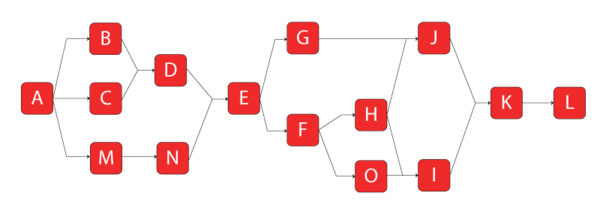The system reliability for this system (computed using BlockSim) is shown next. The first solution is provided using BlockSim's symbolic solution. In symbolic mode, BlockSim breaks the equation into segments, identified by tokens, that need to be substituted into the final system equation for a complete solution. This creates algebraic solutions that are more compact than if the substitutions were made.

\displaystyle \begin{align} {{R}_{System}}= & D2\cdot D3\cdot {{R}_{L}} \\ D3= & +{{R}_{K}}\cdot IK \\ IK= & +{{R}_{I}}\cdot {{R}_{J}}\cdot {{R}_{O}}\cdot {{R}_{G}}\cdot {{R}_{F}}\cdot {{R}_{H}}-{{R}_{I}}\cdot {{R}_{J}}\cdot {{R}_{O}}\cdot {{R}_{G}}\cdot {{R}_{F}} \\ & -{{R}_{I}}\cdot {{R}_{J}}\cdot {{R}_{F}}\cdot {{R}_{H}}-{{R}_{I}}\cdot {{R}_{O}}\cdot {{R}_{F}}\cdot {{R}_{H}} \\ & -{{R}_{J}}\cdot {{R}_{G}}\cdot {{R}_{F}}\cdot {{R}_{H}}+{{R}_{I}}\cdot {{R}_{O}}\cdot {{R}_{F}} \\ & +{{R}_{I}}\cdot {{R}_{F}}\cdot {{R}_{H}}+{{R}_{J}}\cdot {{R}_{F}}\cdot {{R}_{H}}+{{R}_{J}}\cdot {{R}_{G}} \\ D2 = & +{{R}_{A}}\cdot {{R}_{E}}\cdot IE \\ IE = & -D1\cdot {{R}_{M}}\cdot {{R}_{N}}+{{R}_{M}}\cdot {{R}_{N}}+D1 \\ D1 = & +{{R}_{D}}\cdot ID \\ ID = & -{{R}_{B}}\cdot {{R}_{C}}+{{R}_{B}}+{{R}_{C}} \end{align}\,\!

Substituting the terms yields:

\displaystyle \begin{align} {{R}_{System}}= & {{R}_{A}}\cdot {{R}_{E}}\cdot {{R}_{L}}\cdot {{R}_{K}} \\ & \cdot \{({{R}_{D}}\cdot {{R}_{B}}\cdot {{R}_{C}}+{{R}_{B}}+{{R}_{C}})\cdot {{R}_{M}}\cdot {{R}_{N}} \\ & +{{R}_{M}}\cdot {{R}_{N}}-{{R}_{D}}\cdot {{R}_{B}}\cdot {{R}_{C}}+{{R}_{B}}+{{R}_{C}}\} \\ & \cdot \{{{R}_{I}}\cdot {{R}_{J}}\cdot {{R}_{O}}\cdot {{R}_{G}}\cdot {{R}_{F}}\cdot {{R}_{H}}-{{R}_{I}}\cdot {{R}_{J}}\cdot {{R}_{O}}\cdot {{R}_{G}}\cdot {{R}_{F}} \\ & -{{R}_{I}}\cdot {{R}_{J}}\cdot {{R}_{F}}\cdot {{R}_{H}}-{{R}_{I}}\cdot {{R}_{O}}\cdot {{R}_{F}}\cdot {{R}_{H}} \\ & -{{R}_{J}}\cdot {{R}_{G}}\cdot {{R}_{F}}\cdot {{R}_{H}}+{{R}_{I}}\cdot {{R}_{O}}\cdot {{R}_{F}} \\ & +{{R}_{I}}\cdot {{R}_{F}}\cdot {{R}_{H}}+{{R}_{J}}\cdot {{R}_{F}}\cdot {{R}_{H}}+{{R}_{J}}\cdot {{R}_{G}}\} \end{align}\,\!

BlockSim's automatic algebraic simplification would yield the following format for the above solution:

\displaystyle \begin{align} {{R}_{System}}= & (({{R}_{A}}\cdot {{R}_{E}}(-({{R}_{D}}(-{{R}_{B}}\cdot {{R}_{C}}+{{R}_{B}}+{{R}_{C}})){{R}_{M}}\cdot {{R}_{N}} \\ & +{{R}_{M}}\cdot {{R}_{N}} \\ & +({{R}_{D}}(-{{R}_{B}}\cdot {{R}_{C}}+{{R}_{B}}+{{R}_{C}})))) \\ & ({{R}_{K}}({{R}_{I}}\cdot {{R}_{J}}\cdot {{R}_{O}}\cdot {{R}_{G}}\cdot {{R}_{F}}\cdot {{R}_{H}} \\ & -{{R}_{I}}\cdot {{R}_{J}}\cdot {{R}_{O}}\cdot {{R}_{G}}\cdot {{R}_{F}}-{{R}_{I}}\cdot {{R}_{J}}\cdot {{R}_{F}}\cdot {{R}_{H}} \\ & -{{R}_{I}}\cdot {{R}_{O}}\cdot {{R}_{F}}\cdot {{R}_{H}}-{{R}_{J}}\cdot {{R}_{G}}\cdot {{R}_{F}}\cdot {{R}_{H}} \\ & +RI\cdot {{R}_{O}}\cdot {{R}_{F}} \\ & +{{R}_{I}}\cdot {{R}_{F}}\cdot {{R}_{H}}+{{R}_{J}}\cdot {{R}_{F}}\cdot {{R}_{H}}+{{R}_{J}}\cdot {{R}_{G}})){{R}_{L}}) \ \end{align}\,\!

In this equation, each $\displaystyle {{R}_{i}}\,\!$ represents the reliability function of a block. For example, if $\displaystyle {{R}_{A}}\,\!$ has a Weibull distribution, then each $\displaystyle {{R}_{A}}(t)={{e}^{-{{\left( \tfrac{t}{{{\eta }_{A}}} \right)}^{{{\beta }_{A}}}}}}\,\!$ and so forth. Substitution of each component's reliability function in the last $\displaystyle {{R}_{System}}\,\!$ equation above will result in an analytical expression for the system reliability as a function of time, or $\displaystyle {{R}_{s}}(t)\,\!$ , which is the same as $\displaystyle (1-cd{{f}_{System}}).\,\!$

## Obtaining Other Functions of Interest

Once the system reliability equation (or the cumulative density function, cdf) has been determined, other functions and metrics of interest can be derived.

Consider the following simple system:Furthermore, assume that component 1 follows an exponential distribution with a mean of 10,000 ($\displaystyle \mu =10,000,\,\!$ $\displaystyle \lambda =1/10,000)\,\!$ and component 2 follows a Weibull distribution with $\displaystyle \beta =6\,\!$ and $\displaystyle \eta =10,000\,\!$ . The reliability equation of this system is:

\displaystyle \begin{align} {{R}_{S}}(t)= & {{R}_{1}}(t)\cdot {{R}_{2}}(t) \\ = & {{e}^{-\lambda t}}\cdot {{e}^{-{{\left( \tfrac{t}{\eta } \right)}^{\beta }}}} \\ = & {{e}^{-\tfrac{1}{10,000}t}}\cdot {{e}^{-{{\left( \tfrac{t}{10,000} \right)}^{6}}}} \ \end{align}\,\!

The system cdf is:

\displaystyle \begin{align} {{F}_{S}}(t)= & 1-({{R}_{1}}(t)\cdot {{R}_{2}}(t)) \\ = & 1-\left( {{e}^{-\lambda t}}\cdot {{e}^{-{{\left( \tfrac{t}{\eta } \right)}^{\beta }}}} \right) \\ = & 1-\left( {{e}^{-\tfrac{1}{10,000}t}}\cdot {{e}^{-{{\left( \tfrac{t}{10,000} \right)}^{6}}}} \right) \end{align}\,\!

### System pdf

Once the equation for the reliability of the system has been obtained, the system's pdf can be determined. The pdf is the derivative of the reliability function with respect to time or:

$\displaystyle {{f}_{s}}(t)=-\frac{d({{R}_{s}}(t))}{dt} \ \,\!$

For the system shown above, this is:

\displaystyle \begin{align} {{f}_{s}}(t)= & -\frac{d}{dt}\left( {{e}^{-\tfrac{1}{10,000}t}}\cdot {{e}^{-{{\left( \tfrac{t}{10,000} \right)}^{6}}}} \right) \\ = & -\frac{d}{dt}\left( {{e}^{-\tfrac{1}{10,000}t}} \right)\cdot {{e}^{-{{\left( \tfrac{t}{10,000} \right)}^{6}}}} \\ & +{{e}^{-\tfrac{1}{10,000}t}}\left[ -\frac{d}{dt}\left( {{e}^{-{{\left( \tfrac{t}{10,000} \right)}^{6}}}} \right) \right] \\ = & {{f}_{1}}(t)\cdot {{R}_{2}}(t)+{{f}_{2}}(t)\cdot {{R}_{1}}(t) \end{align}\,\!

The next figure shows a plot of the pdf equation.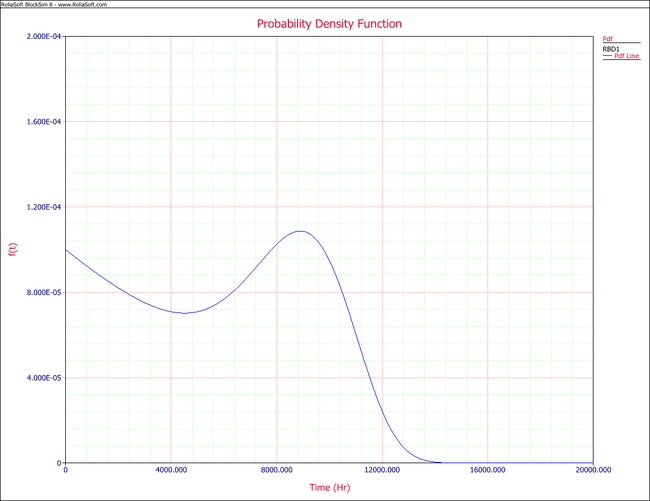### Conditional Reliability

Conditional reliability is the probability of a system successfully completing another mission following the successful completion of a previous mission. The time of the previous mission and the time for the mission to be undertaken must be taken into account for conditional reliability calculations. The system's conditional reliability function is given by:

$\displaystyle R(t|T)=\frac{R(T+t)}{R(T)}\,\!$

Equation above gives the reliability for a new mission of duration $\displaystyle t\,\!$ having already accumulated $\displaystyle T\,\!$ hours of operation up to the start of this new mission. The system is evaluated to assure that it will start the next mission successfully.

For the simple two-component system, the reliability for mission of $\displaystyle t\,\!$ = 1000 hours, having an age of $\displaystyle T\,\!$ = 500 hours, is:

\displaystyle \begin{align} {{R}_{S}}(t=1000|T=500)= & \frac{R(T+t)}{R(T)} \\ = & \frac{R(1500)}{R(500)} \\ = & \frac{{{e}^{-\tfrac{1500}{10,000}}}\cdot {{e}^{-{{\left( \tfrac{1500}{10,000} \right)}^{6}}}}}{{{e}^{-\tfrac{500}{10,000}t}}\cdot {{e}^{-{{\left( \tfrac{500}{10,000} \right)}^{6}}}}} \\ = & 0.9048=90.48% \end{align}\,\!

### Conditional Reliability for Components

Now in this formulation, it was assumed that the accumulated age was equivalent for both units. That is, both started life at zero and aged to 500. It is possible to consider an individual component that has already accumulated some age (used component) in the same formulation. To illustrate this, assume that component 2 started life with an age of T = 100. Then the reliability equation of the system, as given in $\displaystyle {{R}_{S}}(t)= {{R}_{1}}(t)\cdot {{R}_{2}}(t)\,\!$ , would need to be modified to include a conditional term for 2, or:

$\displaystyle {{R}_{S}}(t)={{R}_{1}}(t)\cdot \frac{{{R}_{2}}({{T}_{2}}+t)}{{{R}_{2}}({{T}_{2}})} \ \,\!$

In BlockSim, the start age input box may be used to specify a starting age greater than zero.

### System Failure Rate

Once the distribution of the system has been determined, the failure rate can also be obtained by dividing the pdf by the reliability function:

$\displaystyle {{\lambda }_{s}}\left( t \right)=\frac{{{f}_{s}}\left( t \right)}{{{R}_{s}}\left( t \right)} \ \,\!$

For the simple two-component system:

\displaystyle \begin{align} {{\lambda }_{s}}\left( t \right)= & \frac{-\tfrac{d}{dt}\left( {{e}^{-\tfrac{1}{10,000}t}}\cdot {{e}^{-{{\left( \tfrac{t}{10,000} \right)}^{6}}}} \right)}{{{e}^{-\tfrac{1}{10,000}t}}\cdot {{e}^{-{{\left( \tfrac{t}{10,000} \right)}^{6}}}}} \\ = & \frac{-\tfrac{d}{dt}\left( {{e}^{-\tfrac{1}{10,000}t}} \right)}{{{e}^{-\tfrac{1}{10,000}t}}}+\frac{-\tfrac{d}{dt}\left( {{e}^{-{{\left( \tfrac{t}{10,000} \right)}^{6}}}} \right)}{{{e}^{-{{\left( \tfrac{t}{10,000} \right)}^{6}}}}} \\ = & \frac{{{f}_{1}}}{{{R}_{1}}}+\frac{{{f}_{2}}}{{{R}_{2}}} \\ = & {{\lambda }_{1}}+{{\lambda }_{2}} \ \end{align}\,\!

The following figure shows a plot of the equation.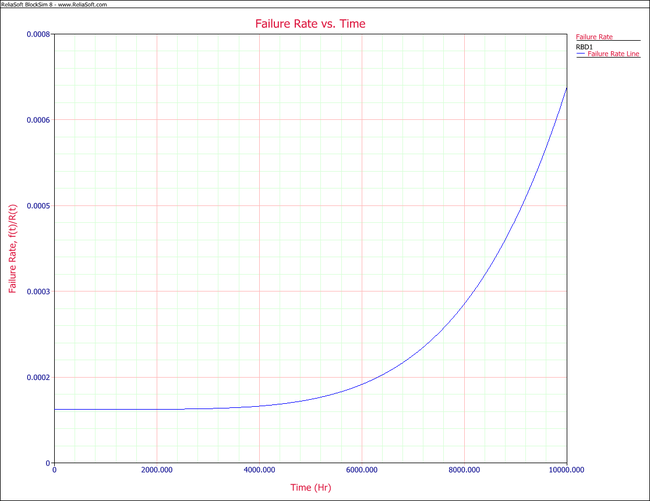BlockSim uses numerical methods to estimate the failure rate. It should be pointed out that as $\displaystyle t\to \infty \,\!$ , numerical evaluation of the first equation above is constrained by machine numerical precision. That is, there are limits as to how large $\displaystyle t\,\!$ can get before floating point problems arise. For example, at $\displaystyle t=5,000,000\,\!$ both numerator and denominator will tend to zero (e.g., $\displaystyle {{e}^{-\tfrac{5,000,000}{10,000}}}=7.1245\times {{10}^{-218}}\,\!$ ). As these numbers become very small they will start looking like a zero to the computer, or cause a floating point error, resulting in a $\displaystyle \tfrac{0}{0}\,\!$ or $\displaystyle \tfrac{X}{0}\,\!$ operation. In these cases, BlockSim will return a value of "$\displaystyle N/A\,\!$ " for the result. Obviously, this does not create any practical constraints.

### System Mean Life (Mean Time To Failure)

The mean life (or mean time to failure, MTTF) can be obtained by integrating the system reliability function from zero to infinity:

$\displaystyle MTTF=\int_{0}^{\infty }{{R}_{s}}\left( t \right)dt \ \,\!$

The mean time is a performance index and does not provide any information about the behavior of the failure distribution of the system.

For the simple two-component system:

\displaystyle \begin{align} MTTF= & \int_{0}^{\infty }\left( {{e}^{-\tfrac{1}{10,000}t}}\cdot {{e}^{-{{\left( \tfrac{t}{10,000} \right)}^{6}}}} \right)dt \\ = & 5978.9 \end{align}\,\!

### Warranty Period and BX Life

Sometimes it is desirable to know the time value associated with a certain reliability. Warranty periods are often calculated by determining what percentage of the failure population can be covered financially and estimating the time at which this portion of the population will fail. Similarly, engineering specifications may call for a certain BX life, which also represents a time period during which a certain proportion of the population will fail. For example, the B10 life is the time in which 10% of the population will fail. This is obtained by setting $\displaystyle {{R}_{S}}(t)\,\!$ to the desired value and solving for $\displaystyle t.\,\!$

For the simple two-component system:

$\displaystyle {{R}_{s}}\left( t \right)={{e}^{-\tfrac{1}{10,000}t}}\cdot {{e}^{-{{\left( \tfrac{t}{10,000} \right)}^{6}}}}\,\!$

To compute the time by which reliability would be equal to 90%, equation above is recast as follows and solved for $\displaystyle t.\,\!$

$\displaystyle 0.90={{e}^{-\tfrac{1}{10,000}t}}\cdot {{e}^{-{{\left( \tfrac{t}{10,000} \right)}^{6}}}}\,\!$

In this case, $\displaystyle t=1053.59\,\!$ . Equivalently, the B10 life for this system is also 1053.59. Except for some trivial cases, a closed form solution for $\displaystyle t\,\!$ cannot be obtained. Thus, it is necessary to solve for $\displaystyle t\,\!$ using numerical methods. BlockSim uses numerical methods.

### Examples

Components in Series

Consider a system consisting of 3 exponential units, connected in series, with the following failure rates (in failures per hour): $\displaystyle {{\lambda }_{1}} = 0.0002\,\!$ , $\displaystyle {{\lambda }_{2}} = 0.0005\,\!$ and $\displaystyle {{\lambda }_{3}} = 0.0001\,\!$ .

• Obtain the reliability equation for the system.
• What is the reliability of the system after 150 hours of operation?
• Obtain the system's pdf.
• Obtain the system's failure rate equation.
• What is the MTTF for the system?
• What should the warranty period be for a 90% reliability?

Solution

The analytical expression for the reliability of the system is given by:

\displaystyle \begin{align} {{R}_{s}}(t)= & {{R}_{1}}(t)\cdot {{R}_{2}}(t)\cdot {{R}_{3}}(t) \\ = & {{e}^{-{{\lambda }_{1}}t}}\cdot {{e}^{-{{\lambda }_{2}}t}}\cdot {{e}^{-{{\lambda }_{1}}t}} \\ = & {{e}^{-({{\lambda }_{1}}+{{\lambda }_{2}}+{{\lambda }_{3}})t}} \end{align}\,\!

At 150 hours of operation, the reliability of the system is:

\displaystyle \begin{align} {{R}_{s}}(t)= & {{e}^{-(0.0002+0.0005+0.0001)150}} \\ = & 0.8869\text{ or }88.69% \end{align}\,\!

In order to obtain the system's pdf, the derivative of the reliability equation given in the first equation above is taken with respect to time, or:

\displaystyle \begin{align} {{f}_{s}}(t)= & -\frac{d[{{R}_{s}}(t)]}{dt} \\ = & -\frac{d\left[ {{e}^{-({{\lambda }_{1}}+{{\lambda }_{2}}+{{\lambda }_{3}})t}} \right]}{dt} \\ = & ({{\lambda }_{1}}+{{\lambda }_{2}}+{{\lambda }_{3}})\cdot {{e}^{-({{\lambda }_{1}}+{{\lambda }_{2}}+{{\lambda }_{3}})t}} \end{align}\,\!

The system's failure rate can now be obtained simply by dividing the system's pdf given in the equation above by the system's reliability function given in the first equation above, and:

\displaystyle \begin{align} {{\lambda }_{s}}\left( t \right)= & \frac{{{f}_{s}}\left( t \right)}{{{R}_{s}}\left( t \right)} \\ = & \frac{({{\lambda }_{1}}+{{\lambda }_{2}}+{{\lambda }_{3}})\cdot {{e}^{-({{\lambda }_{1}}+{{\lambda }_{2}}+{{\lambda }_{3}})t}}}{{{e}^{-({{\lambda }_{1}}+{{\lambda }_{2}}+{{\lambda }_{3}})t}}} \\ = & ({{\lambda }_{1}}+{{\lambda }_{2}}+{{\lambda }_{3}}) \\ = & 0.0008\text{ }fr/hr \end{align}\,\!

Combining $\displaystyle MTTF=\int_{0}^{\infty }{{R}_{s}}\left( t \right)dt \,\!$ and the first equation above, the system's MTTF can be obtained:

\displaystyle \begin{align} MTTF= & \int_{0}^{\infty }{{R}_{s}}\left( t \right)dt \\ = & \int_{0}^{\infty }{{e}^{-({{\lambda }_{1}}+{{\lambda }_{2}}+{{\lambda }_{3}})t}}dt \\ = & \frac{1}{({{\lambda }_{1}}+{{\lambda }_{2}}+{{\lambda }_{3}})} \\ = & 1250\text{ }hr \end{align}\,\!

Solving the first equation above with respect to time will yield the corresponding warranty period for a 90% reliability. In this case, the system reliability equation is simple and a closed form solution exists. The warranty time can now be found by solving:

\displaystyle \begin{align} t= & -\frac{\ln (R)}{{{\lambda }_{1}}+{{\lambda }_{2}}+{{\lambda }_{3}}} \\ = & -\frac{\ln (0.9)}{0.0008} \\ = & 131.7\text{ }hr \end{align}\,\!

Thus, the warranty period should be 132 hours.

Components in Parallel

Consider the system shown next.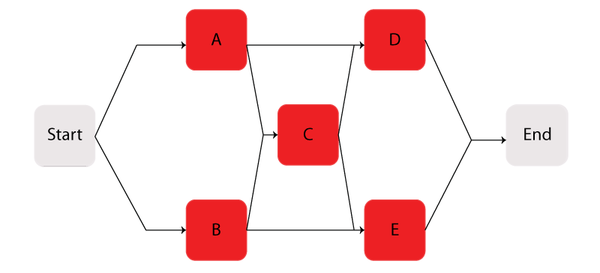Components $\displaystyle A\,\!$ through $\displaystyle E\,\!$ are Weibull distributed with $\displaystyle \beta =1.2\,\!$ and $\displaystyle \eta =1230\,\!$ hours. The starting and ending blocks cannot fail.

Determine the following:

• The reliability equation for the system and its corresponding plot.
• The system's pdf and its corresponding plot.
• The system's failure rate equation and the corresponding plot.
• The MTTF.
• The warranty time for a 90% reliability.
• The reliability for a 200-hour mission, if it is known that the system has already successfully operated for 200 hours.

Solution

The first step is to obtain the reliability function for the system. The methods described in the RBDs and Analytical System Reliability chapter can be employed, such as the event space or path-tracing methods. Using BlockSim, the following reliability equation is obtained:

\displaystyle \begin{align} {{R}_{s}}(t)= & ({{R}_{Start}}\cdot {{R}_{End}}(2{{R}_{A}}\cdot {{R}_{D}}\cdot {{R}_{C}}\cdot {{R}_{B}}\cdot {{R}_{E}} \\ & -{{R}_{A}}\cdot {{R}_{D}}\cdot {{R}_{C}}\cdot {{R}_{B}}-{{R}_{A}}\cdot {{R}_{D}}\cdot {{R}_{C}}\cdot {{R}_{E}} \\ & -{{R}_{A}}\cdot {{R}_{D}}\cdot {{R}_{B}}\cdot {{R}_{E}}-{{R}_{A}}\cdot {{R}_{C}}\cdot {{R}_{B}}\cdot {{R}_{E}} \\ & -{{R}_{D}}\cdot {{R}_{C}}\cdot {{R}_{B}}\cdot {{R}_{E}}+{{R}_{A}}\cdot {{R}_{C}}\cdot {{R}_{E}} \\ & +{{R}_{D}}\cdot {{R}_{C}}\cdot {{R}_{B}}+{{R}_{A}}\cdot {{R}_{D}}+{{R}_{B}}\cdot {{R}_{E}})) \end{align}\,\!

Note that since the starting and ending blocks cannot fail, $\displaystyle {{R}_{Start}}=1\,\!$ and $\displaystyle {{R}_{End}}=1,\,\!$ the equation above can be reduced to:

\displaystyle \begin{align} {{R}_{s}}(t)= & 2\cdot {{R}_{A}}\cdot {{R}_{D}}\cdot {{R}_{C}}\cdot {{R}_{B}}\cdot {{R}_{E}} \\ & -{{R}_{A}}\cdot {{R}_{D}}\cdot {{R}_{C}}\cdot {{R}_{B}}-{{R}_{A}}\cdot {{R}_{D}}\cdot {{R}_{C}}\cdot {{R}_{E}} \\ & -{{R}_{A}}\cdot {{R}_{D}}\cdot {{R}_{B}}\cdot {{R}_{E}}-{{R}_{A}}\cdot {{R}_{C}}\cdot {{R}_{B}}\cdot {{R}_{E}} \\ & -{{R}_{D}}\cdot {{R}_{C}}\cdot {{R}_{B}}\cdot {{R}_{E}}+{{R}_{A}}\cdot {{R}_{C}}\cdot {{R}_{E}} \\ & +{{R}_{D}}\cdot {{R}_{C}}\cdot {{R}_{B}}+{{R}_{A}}\cdot {{R}_{D}}+{{R}_{B}}\cdot {{R}_{E}} \end{align}\,\!

where $\displaystyle {{R}_{A}}\,\!$ is the reliability equation for Component A, or:

$\displaystyle {{R}_{A}}(t)={{e}^{-{{\left( \tfrac{t}{{{\eta }_{A}}} \right)}^{{{\beta }_{A}}}}}}\,\!$

$\displaystyle {{R}_{B}}\,\!$ is the reliability equation for Component $\displaystyle B\,\!$ , etc.

Since the components in this example are identical, the system reliability equation can be further reduced to:

\displaystyle \begin{align} {{R}_{s}}(t)=2R{{(t)}^{2}}+2R{{(t)}^{3}}-5R{{(t)}^{4}}+2R{{(t)}^{5}} \end{align}\,\!

Or, in terms of the failure distribution:

$\displaystyle {{R}_{s}}(t)=2\cdot {{e}^{-2{{\left( \tfrac{t}{\eta } \right)}^{\beta }}}}+2\cdot {{e}^{-3{{\left( \tfrac{t}{\eta } \right)}^{\beta }}}}-5\cdot {{e}^{-4{{\left( \tfrac{t}{\eta } \right)}^{\beta }}}}+2\cdot {{e}^{-5{{\left( \tfrac{t}{\eta } \right)}^{\beta }}}}\,\!$

The corresponding plot is given in the following figure.In order to obtain the system's pdf, the derivative of the reliability equation given above is taken with respect to time, resulting in:

\displaystyle \begin{align} {{f}_{s}}(t)= & 4\cdot \frac{\beta }{\eta }{{\left( \frac{t}{\eta } \right)}^{\beta -1}}{{e}^{-2{{\left( \tfrac{t}{\eta } \right)}^{\beta }}}}+6\cdot \frac{\beta }{\eta }{{\left( \frac{t}{\eta } \right)}^{\beta -1}}{{e}^{-3{{\left( \tfrac{t}{\eta } \right)}^{\beta }}}} \\ & -20\cdot \frac{\beta }{\eta }{{\left( \frac{t}{\eta } \right)}^{\beta -1}}{{e}^{-4{{\left( \tfrac{t}{\eta } \right)}^{\beta }}}}+10\cdot \frac{\beta }{\eta }{{\left( \frac{t}{\eta } \right)}^{\beta -1}}{{e}^{-5{{\left( \tfrac{t}{\eta } \right)}^{\beta }}}} \end{align}\,\!

The pdf can now be plotted for different time values, $\displaystyle t\,\!$ , as shown in the following figure.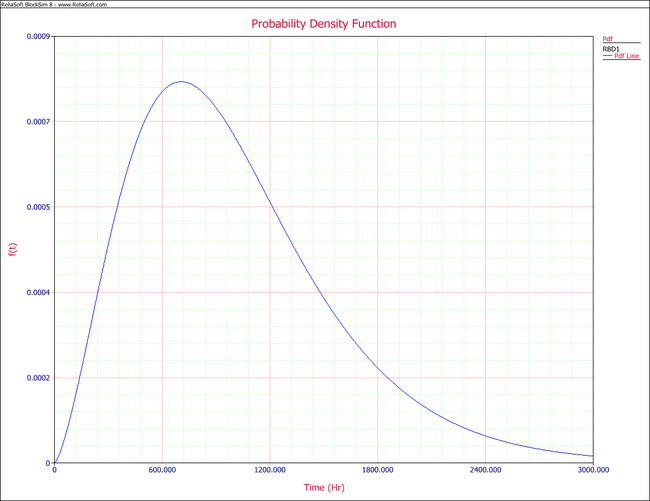The system's failure rate can be obtained by dividing the system's pdf, given in equation above, by the system's reliability function given in

\displaystyle \begin{align} {{R}_{s}}(t)= & 2\cdot {{e}^{-2{{\left( \tfrac{t}{\eta } \right)}^{\beta }}}}+2\cdot {{e}^{-3{{\left( \tfrac{t}{\eta } \right)}^{\beta }}}}-5\cdot {{e}^{-4{{\left( \tfrac{t}{\eta } \right)}^{\beta }}}}+2\cdot {{e}^{-5{{\left( \tfrac{t}{\eta } \right)}^{\beta }}}}\end{align}\,\! , or:
\displaystyle \begin{align} {{\lambda }_{s}}(t)= & \frac{4\cdot \tfrac{\beta }{\eta }{{\left( \tfrac{t}{\eta } \right)}^{\beta -1}}{{e}^{-2{{\left( \tfrac{t}{\eta } \right)}^{\beta }}}}+6\cdot \tfrac{\beta }{\eta }{{\left( \tfrac{t}{\eta } \right)}^{\beta -1}}{{e}^{-3{{\left( \tfrac{t}{\eta } \right)}^{\beta }}}}}{2\cdot {{e}^{-2{{\left( \tfrac{t}{\eta } \right)}^{\beta }}}}+2\cdot {{e}^{-3{{\left( \tfrac{t}{\eta } \right)}^{\beta }}}}-5\cdot {{e}^{-4{{\left( \tfrac{t}{\eta } \right)}^{\beta }}}}+2\cdot {{e}^{-5{{\left( \tfrac{t}{\eta } \right)}^{\beta }}}}} \\ & +\frac{-20\cdot \tfrac{\beta }{\eta }{{\left( \tfrac{t}{\eta } \right)}^{\beta -1}}{{e}^{-4{{\left( \tfrac{t}{\eta } \right)}^{\beta }}}}+10\cdot \tfrac{\beta }{\eta }{{\left( \tfrac{t}{\eta } \right)}^{\beta -1}}{{e}^{-5{{\left( \tfrac{t}{\eta } \right)}^{\beta }}}}}{2\cdot {{e}^{-2{{\left( \tfrac{t}{\eta } \right)}^{\beta }}}}+2\cdot {{e}^{-3{{\left( \tfrac{t}{\eta } \right)}^{\beta }}}}-5\cdot {{e}^{-4{{\left( \tfrac{t}{\eta } \right)}^{\beta }}}}+2\cdot {{e}^{-5{{\left( \tfrac{t}{\eta } \right)}^{\beta }}}}} \end{align}\,\!

The corresponding plot is given below.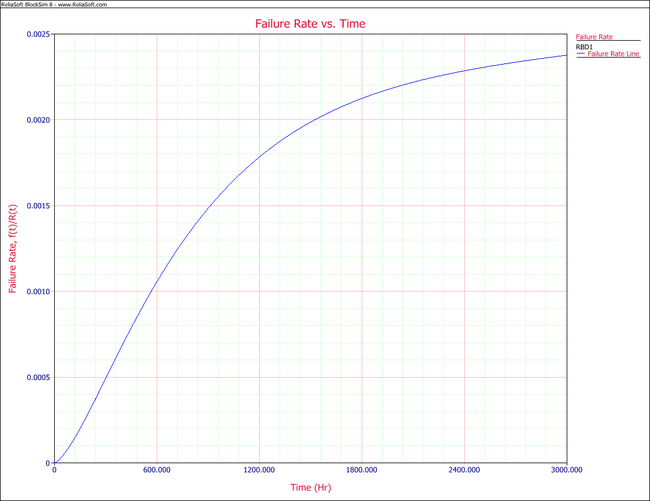The $\displaystyle MTTF\,\!$ of the system is obtained by integrating the system's reliability function given by $\displaystyle {{R}_{s}}(t)=2\cdot {{e}^{-2{{\left( \tfrac{t}{\eta } \right)}^{\beta }}}}+2\cdot {{e}^{-3{{\left( \tfrac{t}{\eta } \right)}^{\beta }}}}-5\cdot {{e}^{-4{{\left( \tfrac{t}{\eta } \right)}^{\beta }}}}+2\cdot {{e}^{-5{{\left( \tfrac{t}{\eta } \right)}^{\beta }}}}\,\!$ from time zero to infinity, as given by $\displaystyle MTTF=\int_{0}^{\infty }{{R}_{s}}\left( t \right)dt \ \,\!$ . Using BlockSim's Analytical QCP, an $\displaystyle MTTF\,\!$ of 1007.8 hours is calculated, as shown in the figure below.The warranty time can be obtained by solving $\displaystyle {{R}_{s}}(t)\,\!$ with respect to time for a system reliability $\displaystyle {{R}_{s}}=0.9\,\!$ . Using the Analytical QCP and selecting the Reliable Life option, a time of 372.72 hours is obtained, as shown in the following figure.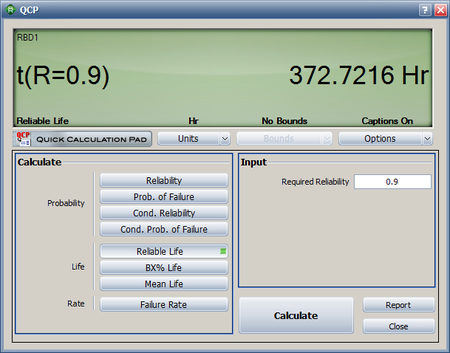Lastly, the conditional reliability can be obtained using $\displaystyle R(t|T)=\frac{R(T+t)}{R(T)}\,\!$ and $\displaystyle {{R}_{s}}(t)=2\cdot {{e}^{-2{{\left( \tfrac{t}{\eta } \right)}^{\beta }}}}+2\cdot {{e}^{-3{{\left( \tfrac{t}{\eta } \right)}^{\beta }}}}-5\cdot {{e}^{-4{{\left( \tfrac{t}{\eta } \right)}^{\beta }}}}+2\cdot {{e}^{-5{{\left( \tfrac{t}{\eta } \right)}^{\beta }}}}\,\!$ , or:

\displaystyle \begin{align} R(200|200)= & \frac{R(400)}{R(200)} \\ = & \frac{0.883825}{0.975321} \\ = & 0.906189 \end{align}\,\!

This can be calculated using BlockSim's Analytical QCP, as shown below.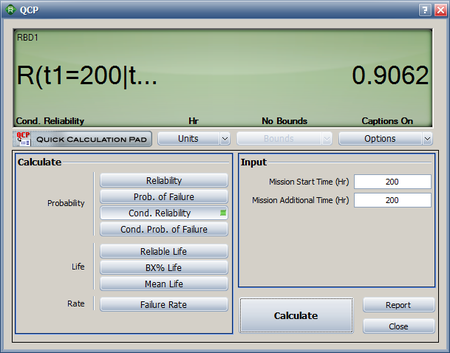## Approximating the System cdf

In many cases, it is valuable to fit a distribution that represents the system's times-to-failure. This can be useful when the system is part of a larger assembly and may be used for repeated calculations or in calculations for other systems. In cases such as this, it can be useful to characterize the system's behavior by fitting a distribution to the overall system and calculating parameters for this distribution. This is equivalent to fitting a single distribution to describe $\displaystyle {{R}_{S}}(t)\,\!$ . In essence, it is like reducing the entire system to a component in order to simplify calculations.

For the system shown below:$\displaystyle {{R}_{S}}(t)={{e}^{-\tfrac{1}{10,000}t}}\cdot {{e}^{-{{\left( \tfrac{t}{10,000} \right)}^{6}}}}\,\!$

To compute an approximate reliability function for this system, $\displaystyle {{R}_{A}}(t)\simeq {{R}_{S}}(t)\,\!$ , one would compute $\displaystyle n\,\!$ pairs of reliability and time values and then fit a single distribution to the data, or:

\displaystyle \begin{align} {{R}_{S}}(t= & 10,396.7)=10% \\ {{R}_{S}}(t= & 9,361.9)=20% \\ & ... \\ {{R}_{S}}(t= & 1,053.6)=90% \end{align}\,\!

A single distribution, $\displaystyle {{R}_{A}}(t)\,\!$ , that approximates $\displaystyle {{R}_{S}}(t)\,\!$ can now be computed from these pairs using life data analysis methods. If using the Weibull++ software, one would enter the values as free-form data.

### Example

Compute a single Weibull distribution approximation for the parallel system in the previous example.

Solution

Assume that the system can be approximated by a 2-parameter Weibull distribution with $\displaystyle \beta =2.02109\,\!$ and $\displaystyle \eta =1123.51\,\!$ . In BlockSim, this is accomplished by representing the entire system as one distribution. To do this, click the Distribution fit area on the diagram's control panel, as shown next.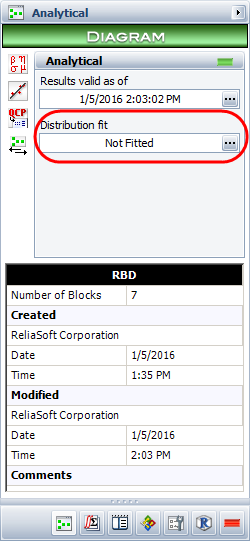This opens the Distribution Estimator window, which allows you to select a distribution to represent the data.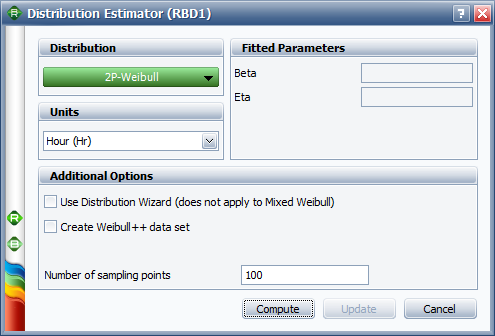When you analyze the diagram, BlockSim will generate a number of system failure times based on the system's reliability function. The system's reliability function can be used to solve for a time value associated with an unreliability value. For example, consider an unreliability value of $\displaystyle F(t)=0.11\,\!$ . Using the system's reliability equation, the corresponding time-to-failure for a 0.11 unreliability is 389.786 hours. The distribution of the generated time values can then be fitted to a probability distribution function.

When enough points have been generated, the selected distribution will be fitted to the generated data set and the distribution's parameters will be calculated. In addition, if ReliaSoft's Weibull++ is installed, the fit of the distribution can be analyzed using a Weibull++ standard folio, as shown in the next figure. It is recommended that the analyst examine the fit to ascertain the applicability of the approximation.## Duty Cycle

Components of a system may not operate continuously during a system's mission, or may be subjected to loads greater or lesser than the rated loads during system operation. To model this, a factor called the Duty Cycle ( $\displaystyle {{d}_{c}}\,\!$ ) is used. The duty cycle may also be used to account for changes in environmental stress, such as temperature changes, that may effect the operation of a component. The duty cycle is a positive value, with a default value of 1 representing continuous operation at rated load, and any values other than 1 representing other load values with respect to the rated load value (or total operating time). A duty cycle value higher than 1 indicates a load in excess of the rated value. A duty cycle value lower than 1 indicates that the component is operating at a load lower than the rated load or not operating continuously during the system's mission. For instance, a duty cycle of 0.5 may be used for a component that operates only half of the time during the system's mission.

The reliability metrics for a component with a duty cycle are calculated as follows. Let $\displaystyle {{d}_{c}}\,\!$ represent the duty cycle during a particular mission of the component, $\displaystyle t\,\!$ represent the mission time and $\displaystyle {t}'\,\!$ represent the accumulated age. Then:

$\displaystyle {t}'={{d}_{c}}\times t\,\!$

The reliability equation for the component is:

$\displaystyle R({t}')=R({{d}_{c}}\times t)\,\!$

The component pdf is:

$\displaystyle f({t}')=-\frac{d(R({t}'))}{dt}=-\frac{d(R({{d}_{c}}\times t))}{dt}={{d}_{c}}f({{d}_{c}}\times t)\,\!$

The failure rate of the component is:

$\displaystyle \lambda ({t}')=\frac{f({t}')}{R({t}')}=\frac{{{d}_{c}}f({{d}_{c}}\times t)}{R({{d}_{c}}\times t)}={{d}_{c}}\lambda ({{d}_{c}}\times t)\,\!$

### Example

Consider a computer system with three components: a processor, a hard drive and a CD drive in series as shown next. Assume that all three components follow a Weibull failure distribution. The processor has the following parameters, $\displaystyle {{\beta }_{1}}=1.5\,\!$ and $\displaystyle {{\eta }_{1}}=5000\,\!$ . For the hard drive, the parameters are $\displaystyle {{\beta }_{2}}=2.5\,\!$ and $\displaystyle {{\eta }_{2}}=3000\,\!$ , and for the CD drive they are $\displaystyle {{\beta }_{3}}=2\,\!$ and $\displaystyle {{\eta }_{3}}=4000\,\!$ . Determine the reliability of the computer system after one year (365 days) of operation, assuming that the CD drive is used only 30% of the time.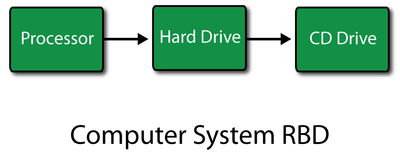Solution

The reliability of the processor after 365 days of operation is given by:

\displaystyle \begin{align} {{R}_{processor}}(365)= & {{e}^{-{{\left( \tfrac{365}{{{\eta }_{1}}} \right)}^{{{\beta }_{1}}}}}} \\ = & {{e}^{-{{\left( \tfrac{365}{5000} \right)}^{1.5}}}} \\ = & 0.9805\text{ or }98.05% \end{align}\,\!

The reliability of the hard drive after 365 days of operation is given by:

\displaystyle \begin{align} {{R}_{harddrive}}(365)= & {{e}^{-{{\left( \tfrac{365}{{{\eta }_{2}}} \right)}^{{{\beta }_{2}}}}}} \\ = & {{e}^{-{{\left( \tfrac{365}{3000} \right)}^{2.5}}}} \\ = & 0.9948\text{ or }99.48% \end{align}\,\!

The reliability of the CD drive after 365 days of operation (taking into account the 30% operation using a duty cycle of 0.3) is given by:

\displaystyle \begin{align} {{R}_{CDdrive}}(365)= & {{e}^{-{{\left( \tfrac{{{d}_{c}}\times 365}{{{\eta }_{3}}} \right)}^{{{\beta }_{3}}}}}} \\ = & {{e}^{-{{\left( \tfrac{0.3\times 365}{4000} \right)}^{2}}}} \\ = & 0.9993\text{ or }99.93% \end{align}\,\!

Thus the reliability of the computer system after 365 days of operation is:

\displaystyle \begin{align} {{R}_{s}}(365)= & {{R}_{processor}}(365)\cdot {{R}_{harddrive}}(365)\cdot {{R}_{CDdrive}}(365) \\ = & 0.9805\cdot 0.9948\cdot 0.9993 \\ = & 0.9747\text{ or }97.47% \end{align}\,\!

The result can also be obtained in BlockSim by creating an RBD of the system and using the Quick Calculation Pad (QCP) to calculate the reliability, as shown in the following figure.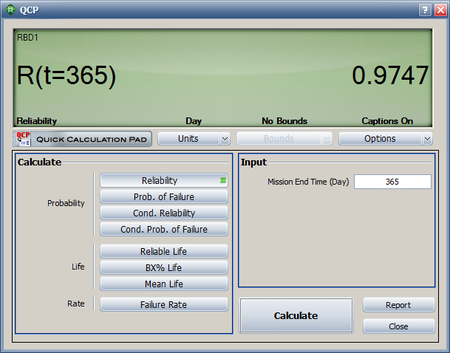As presented in earlier chapters, a reliability block diagram (RBD) allows you to graphically represent how the components within a system are reliability-wise connected. In most cases, independence is assumed across the components within the system. For example, the failure of component A does not affect the failure of component B. However, if a system consists of components that are sharing a load, then the assumption of independence no longer holds true.

If one component fails, then the component(s) that are still operating will have to assume the failed unit's portion of the load. Therefore, the reliabilities of the surviving unit(s) will change. Calculating the system reliability is no longer an easy proposition. In the case of load sharing components, the change of the failure distributions of the surviving components must be known in order to determine the system's reliability.

To illustrate this, consider a system of two units connected reliability-wise in parallel as shown below.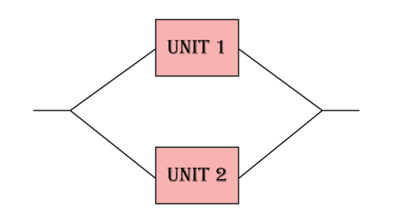Assume that the units must supply an output of 8 volts and that if both units are operational, each unit is to supply 50% of the total output. If one of the units fails, then the surviving unit supplies 100%. Furthermore, assume that having to supply the entire load has a negative impact on the reliability characteristics of the surviving unit.

Because the reliability characteristics of the unit change based on the load it is sharing, a method that can model the effect of the load on life should be used. One way to do this is to use a life distribution along with a life-stress relationship (as discussed in A Brief Introduction to Life-Stress Relationships) for each component. The detailed discussion for this method can be found at Additional Information on Load Sharing. Another simple way is to use the concept of acceleration factors and assume that the load has a linear effect on the failure time. If the load is doubled, then the life of the component will be shortened by half.

For the above load sharing system, the reliability of each component is a function of time and load. For example, for Unit 1, the reliability and the probability density function are:

$\displaystyle {{R}_{1}}(t,{{S}_{1}})\,\!\,\!$ and $\displaystyle {{f}_{1}}(t,{{S}_{1}})\,\!\,\!$

where $\displaystyle {{S}_{1}}\,\!\,\!$ is the load shared by Unit 1 at time t and the total load of the system is $\displaystyle S={{S}_{1}}+{{S}_{2}}\,\!\,\!$ . At the beginning, both units are working. Assume that Unit 1 fails at time x and Unit 2 takes over the entire load. The reliability for Unit 2 at time x is:

\displaystyle \begin{align} & {{R}_{2}}(x,{{S}_{2}})={{R}_{2}}({{t}_{2e}},S) \\ & {{t}_{2e}}=\frac{{{S}_{2}}}{S}x \\ \end{align}\,\!\,\!

$\displaystyle {{t}_{2e}}\,\!\,\!$ is the equivalent time for Unit 2 at time x if it is operated with load S. The equivalent time concept is illustrated in the following plot.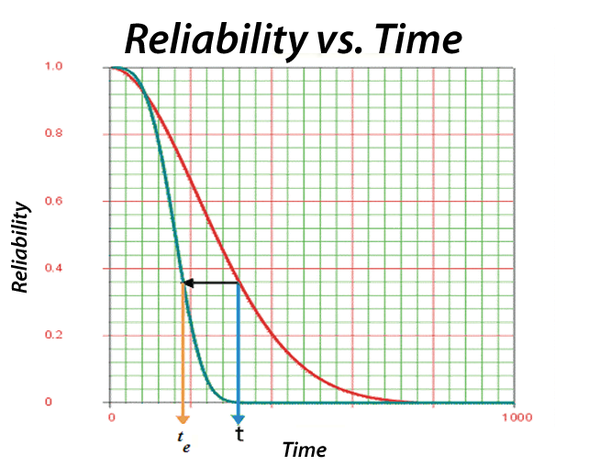The system reliability at time t is:

\displaystyle \begin{align} & R(t)={{R}_{1}}(t,{{S}_{1}})\cdot {{R}_{2}}(t,{{S}_{2}}) \\ & +\int_{0}^{t}{{{f}_{1}}(x,{{S}_{1}})\cdot {{R}_{2}}({{t}_{2e}}+(t-x),S)dx} \\ & +\int_{0}^{t}{{{f}_{2}}(x,{{S}_{2}})\cdot {{R}_{1}}({{t}_{1e}}+(t-x),S)dx} \end{align}\,\!\,\!

In BlockSim, the failure time distribution for each component is defined at the load of S. The reliability function for a component at a given load is calculated as:

$\displaystyle {{R}_{i}}(t,{{S}_{i}})={{R}_{i}}(t\times \frac{{{S}_{i}}}{S},S)\,\!\,\!$
$\displaystyle {{f}_{i}}(t,{{S}_{i}})=\frac{{{S}_{i}}}{S}{{f}_{i}}\left( t\times \frac{{{S}_{i}}}{S},S \right)\,\!\,\!$

From the above equation, it can be seen that the concept used in the calculation for load sharing is the same as the concept used in the calculation for duty cycle.

### Example

In the following load sharing system, Block 1 follows a Weibull failure distribution with$\displaystyle {{\beta }_{1}}=1.5\,\!$ , and$\displaystyle {{\eta }_{1}}=1,000\,\!$ . Block 2 follows a Weibull failure distribution with$\displaystyle {{\beta }_{2}}=2\,\!$ , and$\displaystyle {{\eta }_{2}}=2,000\,\!$ . The load for Block 1 is 1 unit, and for Block 2 it is 3 units. Calculate the system reliability at time 1,500.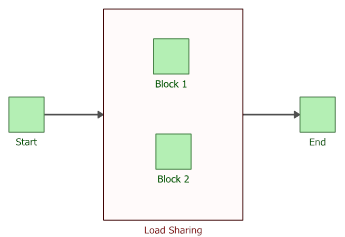Block 1 shares 25% (P1) of the entire load, and Block 2 shares 75% (P2) of it. Therefore, we have the following equations for calculating the system reliability:

$\displaystyle {{R}_{i}}(t,{{S}_{i}})={{R}_{i}}({{P}_{i}}\times t)\,\!$ ,
$\displaystyle {{f}_{i}}(x,{{S}_{i}})={{P}_{i}}{{f}_{i}}({{P}_{i}}\times x)\,\!$

and:

$\displaystyle {{R}_{1}}({{t}_{1e}}+(t-x))={{R}_{1}}({{P}_{1}}x+t-x)={{R}_{1}}(t-{{P}_{2}}x)\,\!$
$\displaystyle {{R}_{2}}({{t}_{2e}}+(t-x))={{R}_{2}}({{P}_{2}}x+t-x)={{R}_{2}}(t-{{P}_{1}}x)\,\!$

Using the above equations in the system reliability function, we get:

\displaystyle \begin{align} & R(t)={{R}_{1}}(t,{{S}_{1}})\cdot {{R}_{2}}(t,{{S}_{2}})+\int_{0}^{t}{{{f}_{1}}(x,{{S}_{1}})\cdot {{R}_{2}}({{t}_{2e}}+(t-x),S)dx} \\ & +\int_{0}^{t}{{{f}_{2}}(x,{{S}_{2}})\cdot {{R}_{1}}({{t}_{1e}}+(t-x),S)dx} \\ & ={{R}_{1}}({{P}_{1}}\times t)\cdot {{R}_{2}}({{P}_{2}}\times t)+\int_{0}^{t}{{{P}_{1}}{{f}_{1}}({{P}_{1}}x)\cdot {{R}_{2}}(t-{{P}_{1}}x)dx} \\ & +\int_{0}^{t}{{{P}_{2}}{{f}_{2}}({{P}_{2}}x)\cdot {{R}_{1}}(t-{{P}_{2}}x)dx} \end{align}\,\!

The calculated system reliability at time 1,500 is 0.8569, as given below.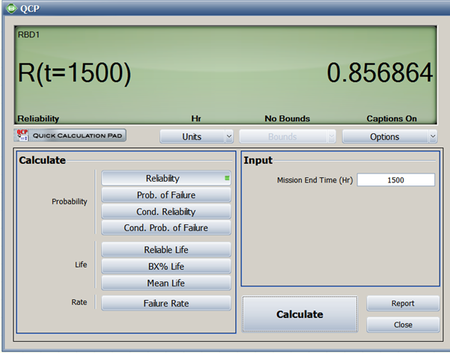## Standby Components

In the previous section, the case of a system with load sharing components was presented. This is a form of redundancy with dependent components. That is, the failure of one component affects the failure of the other(s). This section presents another form of redundancy: standby redundancy. In standby redundancy the redundant components are set to be under a lighter load condition (or no load) while not needed and under the operating load when they are activated.

In standby redundancy the components are set to have two states: an active state and a standby state. Components in standby redundancy have two failure distributions, one for each state. When in the standby state, they have a quiescent (or dormant) failure distribution and when operating, they have an active failure distribution.

In the case that both quiescent and active failure distributions are the same, the units are in a simple parallel configuration (also called a hot standby configuration). When the rate of failure of the standby component is lower in quiescent mode than in active mode, that is called a warm standby configuration. When the rate of failure of the standby component is zero in quiescent mode (i.e., the component cannot fail when in standby), that is called a cold standby configuration.

### Simple Standby Configuration

Consider two components in a standby configuration. Component 1 is the active component with a Weibull failure distribution with parameters $\displaystyle \beta = 1.5\,\!$ and $\displaystyle \eta = 1,000 \,\!$ . Component 2 is the standby component. When Component 2 is operating, it also has a Weibull failure distribution with $\displaystyle \beta = 1.5\,\!$ and $\displaystyle \eta = 1,000 \,\!$ . Furthermore, assume the following cases for the quiescent distribution.

• Case 1: The quiescent distribution is the same as the active distribution (hot standby).
• Case 2: The quiescent distribution is a Weibull distribution with $\displaystyle \beta = 1.5\,\!$ and $\displaystyle \eta = 2000\,\!$ (warm standby).
• Case 3: The component cannot fail in quiescent mode (cold standby).

In this case, the reliability of the system at some time, $\displaystyle t\,\!$ , can be obtained using the following equation:

$\displaystyle R(t)={{R}_{1}}(t)+\underset{0}{\overset{t}{\mathop \int }}\,{{f}_{1}}(x)\cdot {{R}_{2;SB}}(x)\cdot \frac{{{R}_{2;A}}({{t}_{e}}+t-x)}{{{R}_{2;A}}({{t}_{e}})}dx \ \,\!$

where:

• $\displaystyle {{R}_{1}}\,\!$ is the reliability of the active component.
• $\displaystyle {{f}_{1}}\,\!$ is the pdf of the active component.
• $\displaystyle {{R}_{2;SB}}\,\!$ is the reliability of the standby component when in standby mode (quiescent reliability).
• $\displaystyle {{R}_{2;A}}\,\!$ is the reliability of the standby component when in active mode.
• $\displaystyle {{t}_{e}}\,\!$ is the equivalent operating time for the standby unit if it had been operating at an active mode, such that:
\displaystyle \begin{align} {{R}_{2;SB}}(x)={{R}_{2;A}}({{t}_{e}}) \end{align}\,\!

The second equation above can be solved for $\displaystyle {{t}_{e}}\,\!$ and substituted into the first equation above. The following figure illustrates the example as entered in BlockSim using a standby container.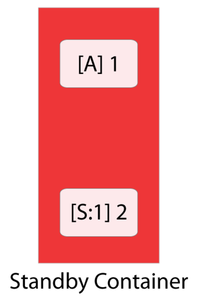The active and standby blocks are within a container, which is used to specify standby redundancy. Since the standby component has two distributions (active and quiescent), the Block Properties window of the standby block has two pages for specifying each one. The following figures illustrate these pages.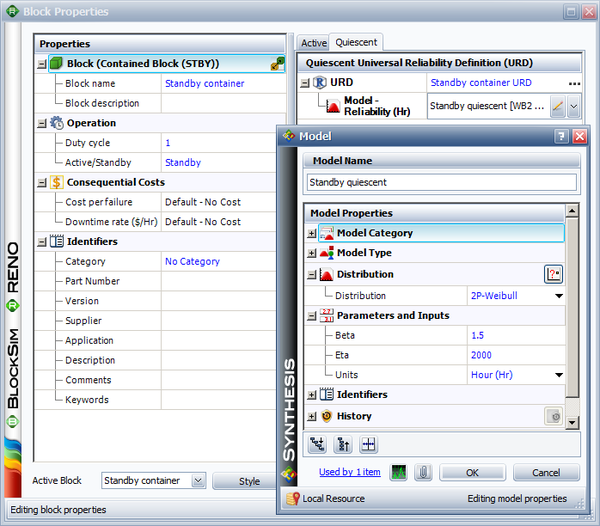The system reliability results for 1000 hours are given in the following table: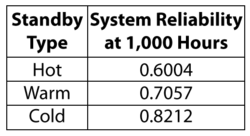Note that even though the $\displaystyle \beta \,\!$ value for the quiescent distribution is the same as in the active distribution, it is possible that the two can be different. That is, the failure modes present during the quiescent mode could be different from the modes present during the active mode. In that sense, the two distribution types can be different as well (e.g., lognormal when quiescent and Weibull when active).

In many cases when considering standby systems, a switching device may also be present that switches from the failed active component to the standby component. The reliability of the switch can also be incorporated into $\displaystyle R(t)={{R}_{1}}(t)+\underset{0}{\overset{t}{\mathop \int }}\,{{f}_{1}}(x)\cdot {{R}_{2;SB}}(x)\cdot \frac{{{R}_{2;A}}({{t}_{e}}+t-x)}{{{R}_{2;A}}({{t}_{e}})}dx \ \,\!$

as presented in the next section.

BlockSim's System Reliability Equation window returns a single token for the reliability of units in a standby configuration. This is the same as the load sharing case presented in the previous section.

### Reliability of Standby Systems with a Switching Device

In many cases when dealing with standby systems, a switching device is present that will switch to the standby component when the active component fails. Therefore, the failure properties of the switch must also be included in the analysis.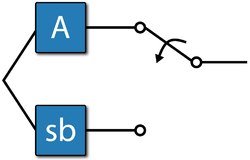In most cases when the reliability of a switch is to be included in the analysis, two probabilities can be considered. The first and most common one is the probability of the switch performing the action (i.e., switching) when requested to do so. This is called Switch Probability per Request in BlockSim and is expressed as a static probability (e.g., 90%). The second probability is the quiescent reliability of the switch. This is the reliability of the switch as it ages (e.g., the switch might wear out with age due to corrosion, material degradation, etc.). Thus it is possible for the switch to fail before the active component fails. However, a switch failure does not cause the system to fail, but rather causes the system to fail only if the switch is needed and the switch has failed. For example, if the active component does not fail until the mission end time and the switch fails, then the system does not fail. However, if the active component fails and the switch has also failed, then the system cannot be switched to the standby component and it therefore fails.

In analyzing standby components with a switching device, either or both failure probabilities (during the switching or while waiting to switch) can be considered for the switch, since each probability can represent different failure modes. For example, the switch probability per request may represent software-related issues or the probability of detecting the failure of an active component, and the quiescent probability may represent wear-out type failures of the switch.

To illustrate the formulation, consider the previous example that assumes perfect switching. To examine the effects of including an imperfect switch, assume that when the active component fails there is a 90% probability that the switch will switch from the active component to the standby component. In addition, assume that the switch can also fail due to a wear-out failure mode described by a Weibull distribution with $\displaystyle \beta = 1.7\,\!$ and $\displaystyle \eta = 5000\,\!$ .

Therefore, the reliability of the system at some time, $\displaystyle t\,\!$ , is given by the following equation.

\displaystyle \begin{align} R(t)= & {{R}_{1}}(t) +\underset{0}{\overset{t}{\mathop \int }}\,\{{{f}_{1}}(x)\cdot {{R}_{2;SB}}(x) \\ & \cdot \frac{{{R}_{2;A}}({{t}_{e}}+t-x)}{{{R}_{2;A}}({{t}_{e}})}\cdot {{R}_{SW;Q}}(x)\cdot {{R}_{SW;REQ}}(x)\}dx \end{align}\,\!

where:

• $\displaystyle {{R}_{1}}\,\!$ is the reliability of the active component.
• $\displaystyle {{f}_{1}}\,\!$ is the pdf of the active component.
• $\displaystyle {{R}_{2;SB}}\,\!$ is the reliability of the standby component when in standby mode (quiescent reliability).
• $\displaystyle {{R}_{2;A}}\,\!$ is the reliability of the standby component when in active mode.
• $\displaystyle {{R}_{SW;Q}}\,\!$ is the quiescent reliability of the switch.
• $\displaystyle {{R}_{SW;REQ}}\,\!$ is the switch probability per request.
• $\displaystyle {{t}_{e}}\,\!$ is the equivalent operating time for the standby unit if it had been operating at an active mode.

This problem can be solved in BlockSim by including these probabilities in the container's properties, as shown in the figures below. In BlockSim, the standby container is acting as the switch.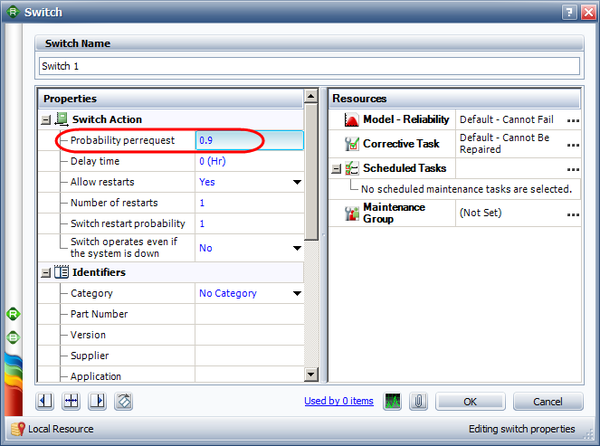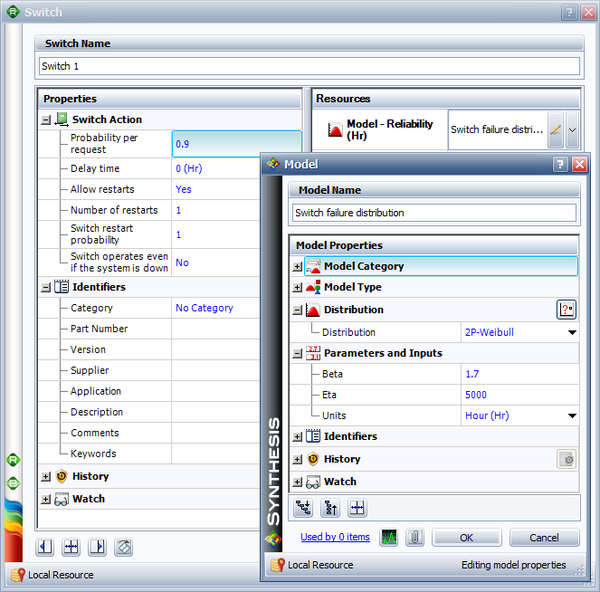Note that there are additional properties that can be specified in BlockSim for a switch, such as Switch Restart Probability, No. of Restarts and Switch Delay Time. In many applications, the switch is re-tested (or re-cycled) if it fails to switch the first time. In these cases, it might be possible that it switches in the second or third, or $\displaystyle {{n}^{th}}\,\!$ attempt.

The Switch Restart Probability specifies each additional attempt's probability of successfully switching and the Finite Restarts specifies the total number of attempts. Note that the Switch Restart Probability specifies the probability of success of each trial (or attempt). The probability of success of $\displaystyle n\,\!$ consecutive trials is calculated by BlockSim using the binomial distribution and this probability is then incorporated into equation above. The Switch Delay Time property is related to repairable systems and is considered in BlockSim only when using simulation. When using the analytical solution (i.e., for a non-repairable system), this property is ignored.

Solving the analytical solution (as given by the above equation), the following results are obtained.

From the table above, it can be seen that the presence of a switching device has a significant effect on the reliability of a standby system. It is therefore important when modeling standby redundancy to incorporate the switching device reliability properties. It should be noted that this methodology is not the same as treating the switching device as another series component with the standby subsystem. This would be valid only if the failure of the switch resulted in the failure of system (e.g., switch failing open). In equation above, the Switch Probability per Request and quiescent probability are present only in the second term of the equation. Treating these two failure modes as a series configuration with the standby subsystem would imply that they are also present when the active component is functioning (i.e., first term of equation above). This is invalid and would result in the underestimation of the reliability of the system. In other words, these two failure modes become significant only when the active component fails.

As an example, and if we consider the warm standby case, the reliability of the system without the switch is 70.57% at 1000 hours. If the system was modeled so that the switching device was in series with the warm standby subsystem, the result would have been:

\displaystyle \begin{align} {{R}_{S}}(1000)= & {{R}_{Standby}}(1000)\cdot {{R}_{sw,Q(1000)}}\cdot {{R}_{sw,req}} \\ = & 0.7057\cdot 0.9372\cdot 0.9 \\ = & 0.5952 \end{align}\,\!

In the case where a switch failure mode causes the standby subsystem to fail, then this mode can be modeled as an individual block in series with the standby subsystem.

### Example

Consider a car with four new tires and a full-size spare. Assume the following failure characteristics:

• The tires follow a Weibull distribution with a $\displaystyle \beta=4\,\!$ and an $\displaystyle \eta = 40,000\,\!$ miles while on the car due to wear.
• The tires also have a probability of failing due to puncture or other causes. For this, assume a constant rate for this occurrence with a probability of 1 every 50,000 miles.
• When not on the car (i.e., is a spare), a tire's probability of failing also has a Weibull distribution with a $\displaystyle \beta = 2\,\!$ and $\displaystyle \eta = 120,000\,\!$ miles.

Assume a mission of 1,000 miles. If a tire fails during this trip, it will be replaced with the spare. However, the spare will not be repaired during the trip. In other words, the trip will continue with the spare on the car and, if the spare fails, the system will fail. Determine the probability of system failure.

Solution

The active failure distribution for the tires are:

• Due to wearout, Weibull $\displaystyle \beta =4\,\!$ and $\displaystyle \eta =40,000\,\!$ miles.
• Due to random puncture, exponential $\displaystyle \mu =50,000.\,\!$
• The quiescent failure distribution is a Weibull distribution with $\displaystyle \beta =2\,\!$ and $\displaystyle \eta =120,000\,\!$ miles.

The block diagram for each tire has two blocks in series, one block representing the wearout mode and the other the random puncture mode, as shown next:There are five tires, four active and one standby (represented in the diagram by a standby container with a 4-out-of-5 requirement), as shown next: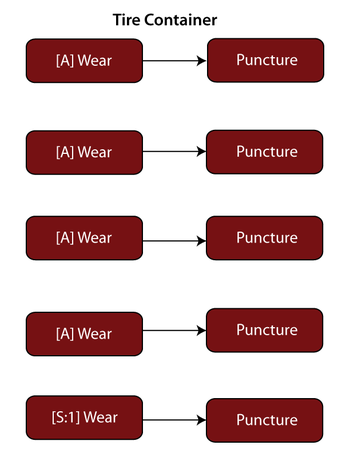For the standby Wear block, set the active failure and the quiescent distributions, but for the Puncture block, set only the active puncture distribution (because the tire cannot fail due to puncture while stored). Using BlockSim, the probability of system failure is found to be 0.003 or 0.3%.